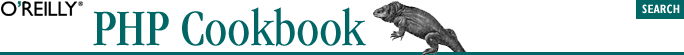home | O'Reilly's CD bookshelfs | FreeBSD | Linux | Cisco | Cisco Exam## 2.7. Generating Biased Random Numbers

### 2.7.3. Discussion

Imagine if instead of an array in which the values are the number of remaining impressions, you have an array of ads in which each ad occurs exactly as many times as its remaining number of impressions. You can simply pick an unweighted random place within the array, and that'd be the ad that shows.

This technique can consume a lot of memory if you have millions of impressions remaining. Instead, you can calculate how large that array would be (by totalling the remaining impressions), pick a random number within the size of the make-believe array, and then go through the array figuring out which ad corresponds to the number you picked. For instance:

```\$ads = array('ford' => 12234, // advertiser, remaining impressions
'att'  => 33424,
'ibm'  => 16823);